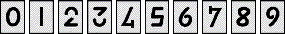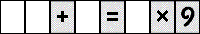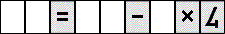2012 U. S. Championship Puzzles

1-2. Math Flip

Fill in the blank spaces in each puzzle with the digits shown below so that the equation is true, and remains true even when viewed upside down. (Note that the 2 and 3 look like one another upside down, as do the 4 and 7, and 6 and 9.) No puzzle contains any digit more than once. Standard order of operations are used: multiplication and division before addition and subtraction, and otherwise left to right.1.2.